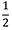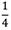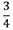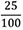## Mathematics 5

### Course Outline

#### Number

• read, write, represent, and describe numbers to 1 000 000 using concrete materials, pictures,  expressions, expanded notation, words, place-value charts, and symbols
• describe numbers to 1 000 000 in two or more parts (partition)
• explore place value (ones, tens, hundreds, thousands, ten thousands, hundred thousands, millions)
• use place-value patterns to understand numbers up to  1 000 000
• compare and order large numbers to 1 000 000
• represent and describe decimal numbers (tenths, hundredths, thousandths) using concrete materials, pictures, money, expressions, words, and symbols
• read, write, and recognize decimal number symbols (to thousandths)
• compare and order decimal numbers (to thousandths)
• represent, interpret, model, and rename fractions using concrete materials, pictures, and symbols
• compare and order fractions (use benchmarks, compare numerators and denominators)
• rename fractions (, andand with denominators of 10, 100, and 1000) as decimals
• rename decimals as fractions (e.g., 0.25 =)
• estimate sums, differences, products, and quotients (involving whole numbers) using appropriate mental mathematics strategies
• estimate the answers to addition and subtraction questions that use decimals to the thousandths
• explain the reasons for our estimates
• choose appropriate estimates
• solve and create addition and subtraction problems that involve whole numbers and decimals
• use personal strategies to calculate sums and differences involving whole numbers and decimals to the thousandths
• represent multiplication and division problems concretely, pictorially, and symbolically
• quickly recall multiplication and division basic facts
• mentally multiply by 10, 100, and 1000 and their multiples
• use mental mathematics strategies to make multiplication more efficient
• continue to use personal strategies to multiply one-, two-, and three-digit numbers by a one-digit number
• use personal strategies to multiply two two-digit numbers
• use personal strategies to divide two- and three-digit numbers by a one-digit number
• solve and create multiplication and division story problems that involve whole numbers
• explain the treatment of a remainder in a division story problem

#### Patterns and Relations (PR)

• identify and describe (orally and in writing) patterns
• identify and describe patterns found in charts and tables using an expression
• use an expression to represent a pattern
• extend an increasing or decreasing pattern and describe how the terms in the pattern change from one step to the next
• determine missing or subsequent elements in a pattern
• identify errors in a pattern
• use a pattern rule to continue a pattern
• use patterns to solve problems
• create a table or chart from a pattern shown concretely
• create a pattern concretely from a table or chart
• use a letter variable to represent the unknown in an addition, subtraction, multiplication, or division problem (e.g., 24 ÷ n = 6, 24 = 6 × n or 24 = 6 × n)
• write an equation with a variable to represent a given story problem
• create a story problem for a given equation with one unknown
• solve a one-step equation with one variable
• represent addition, subtraction, multiplication, and division situations with an equation having one variable

#### Measurement

• draw rectangles for a given perimeter (distance around the outside)
• draw rectangles for a given area
• recognize and demonstrate that different objects can have the same area (inside space)
• recognize and demonstrate that objects with the same area can have different perimeters (distance around the outside)
• determine the shape that will result in the least or the greatest area for a given perimeter
• estimate and measure area in square centimetres and square metres
• solve simple problems involving the perimeter and/or area of polygons
• identify a referent for one millimetre, one centimetre, and one metre
• understand that 10 millimetres is equal to 1 centimetre and 1000 millimetres is equal to 1 metre
• estimate and measure length in millimetres, centimetres, and metres
• determine which unit of measurement is appropriate to use in a given situation
• identify a referent for a cubic centimetre and a cubic metre
• estimate and measure volume (the amount of space occupied by a 3-D object) using standard units (cubic centimetres and cubic metres)
• estimate and then determine the volume of rectangular prisms using centimetre cubes
• construct a rectangular prism for a given volume using cubes
• provide a referent for one litre and one millilitre
• understand that 1000 millilitres is equal to 1 litre
• estimate and measure capacity (the amount a container is capable of holding) using materials that take on the shape of the inside of a container, and using standard units (millilitre and litre)
• solve measurement problems (area, perimeter, length, volume, and capacity)

#### Geometry

• recognize, name, and describe parallel, intersecting, perpendicular, vertical, and horizontal edges and faces on 3-D objects
• recognize, name, and describe parallel, intersecting, perpendicular, vertical, and horizontal sides on 2-D shapes
• draw 2-D shapes with sides that are parallel, intersecting, perpendicular, vertical, and horizontal
• build 3-D objects with edges and faces that are parallel, intersecting, perpendicular, vertical, and horizontal
• identify, name, and sort quadrilaterals according to their attributes
• identify a translation (slide) of a 2-D shape
• identify a rotation (turn) of a 2-D shape
• identify a reflection (flip) of a 2-D shape
• describe the direction and magnitude of a translation (slide)
• describe the direction of turn of a rotation (turn)
• describe the line of reflection (e.g., horizontal, vertical) and the distance from the line of reflection (flip)
• identify transformations (flips, slides, and turns) found in the everyday world
• perform a translation (slide) of a 2-D shape
• perform a rotation (turn) of a 2-D shape
• perform a reflection (flip) of a 2-D shape
• predict the result of a transformation (a flip, a slide, or a turn) and verify the prediction
• identify and sketch right angles
• identify angles that are greater than and less than a right angle

#### Statistics and Probability

• explain the difference between first-hand data and second-hand data